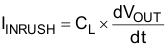JAJSCS0C December   2016  – January 2018

PRODUCTION DATA.

1. 特長
2. アプリケーション
3. 概要
4. 改訂履歴
5. Device Comparison Table
6. Pin Configuration and Functions
7. Specifications
8. Parameter Measurement Information
9. Detailed Description
1. 9.1 Overview
2. 9.2 Functional Block Diagram
3. 9.3 Feature Description
4. 9.4 Device Functional Modes
10. 10Application and Implementation
1. 10.1 Application Information
2. 10.2 ON and OFF Control
3. 10.3 Input Capacitor (Optional)
4. 10.4 Output Capacitor (Optional)
5. 10.5 Typical Application
11. 11Power Supply Recommendations
12. 12Layout
13. 13デバイスおよびドキュメントのサポート
1. 13.1 デバイス・サポート
2. 13.2 ドキュメントのサポート
3. 13.3 ドキュメントの更新通知を受け取る方法
4. 13.4 コミュニティ・リソース
5. 13.5 商標
6. 13.6 静電気放電に関する注意事項
7. 13.7 Glossary
14. 14メカニカル、パッケージ、および注文情報

• DRV|6
• DBV|6
• DRV|6

#### 10.5.2.3 Inrush Current

To determine how much inrush current is caused by the CL capacitor, use Equation 9.

Equation 9.where

• IINRUSH is the amount of inrush caused by CL
• CL is the capacitance on VOUT
• dt is the Output Voltage rise time during the ramp up of VOUT when the device is enabled
• dVOUT is the change in VOUT during the ramp up of VOUT when the device is enabled

The appropriate rise time can be calculated using the design requirements and the inrush current equation. As we calculate the rise time (measured from 10% to 90% of VOUT), we account for this in our dVOUT parameter (80% of VOUT = 9.6 V) as shown in Equation 10 and Equation 11.

Equation 10. 400 mA = 22 µF × 9.6 V/dt
Equation 11. dt = 528 µs

To ensure an inrush current of less than 400 mA, choose a CT value that yields a rise time of more than 528 μs. Consulting Table 2 at VIN = 12 V, CT = 4700 pF provides a typical rise time of 957 μs. Using this rise time and voltage into Equation 9, yields Equation 12 and Equation 13.

Equation 12. IInrush = 22 µF × 9.6 V/ 957 µs
Equation 13. Inrush = 220 mA

An appropriate CL value must be placed on VOUT such that the IMAX and IPLS specifications of the device are not violated.